# Waveform shape & Instantaneous Frequency#

Here we explore how the instantaneous frequency of a signal is related to its waveform shape and how we can directly compare waveform shapes using phase alignment

We will start with some imports emd and by simulating a very simple stationary sine wave signal.

import emd
import numpy as np
from scipy import signal, stats
import matplotlib.pyplot as plt


## Linear & Non-linear Systems#

In this tutorial, we’re going to explore how the instaneous frequency of an oscillatory signal can represent its waveform shape. To do this, we’re going create a sine-wave simulation and modulate by a linear and a non-linear equation. The linear equation simply scales the signal by a defined factor. The non-linear equation also scales the signal but, crucially, has an extra term which distorts the waveform of the oscillation such that it becomes non-sinusoidal.

These equations are implemented as functions below. The equations themselves were defined in equations 50.24 and 50.25 in section 50-6 of Feynman’s Lectures of Physics.

def linear_system(x, K):
""" A linear system which scales a signal by a factor"""
return K * x

def nonlinear_system(x, K, eta=.43, power=2):
""" A non-linear system which scales a signal by a factor introduces a
waveform distortion"""
return K * (x + eta * (x ** power))


## A simple sine-wave#

We will first apply our linear and non-linear equations to a very simple pure-tone oscillation. We define some values below and create a 10 second signal which oscillates at 2Hz.

seconds = 10
f = 2
sample_rate = 512
emd.spectra.frequency_transform
t = np.linspace(0, seconds, seconds*sample_rate)
x = np.cos(2*np.pi*f*t)


We then modulate our signal x by the linear and nonlinear systems.

K = .5  # both systems will scale the signal by 0.5

# Apply the systems to time-series x
x_linear = linear_system(x, K)
x_nonlinear = nonlinear_system(x, K)

# Create a summary plot
plt.figure(figsize=(10, 4))
plt.plot(t, x, 'k:')
plt.plot(t, x_linear)
plt.plot(t, x_nonlinear)
plt.xlim(0, 3)
plt.xlabel('Time (seconds)')
plt.legend(['Original', 'Linear', 'Non-linear'])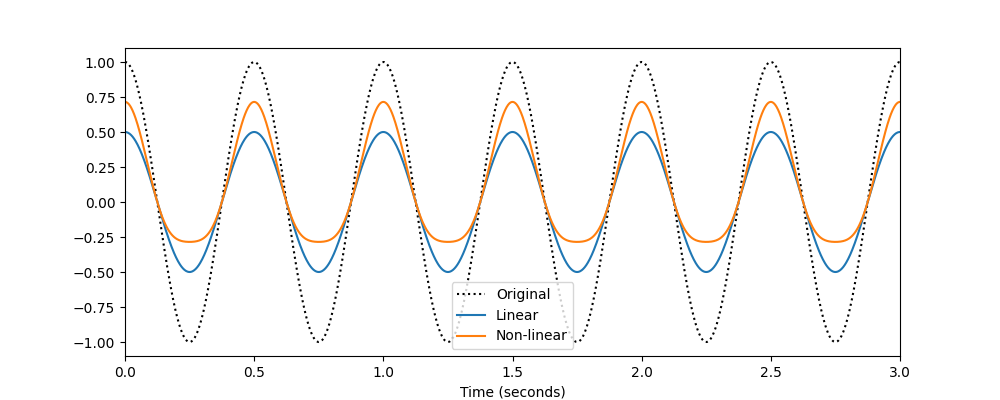<matplotlib.legend.Legend object at 0x7fbf432cc350>


We can see that the output of the linear system returns a scaled sinusoid whilst the nonlinear system outputs a distorted wave. By eye, we can see that the non-linear signal has a sharper peak and a wider trough than the linear system. The next section is going to quantify this distortion using instantanous frequency.

Firstly, we compute the EMD of the linear system using the emd.sift.sift with default argumnts.

# Compute EMD
imf_linear = emd.sift.sift(x_linear)

# Visualise the IMFs
emd.plotting.plot_imfs(imf_linear[:sample_rate*4, :])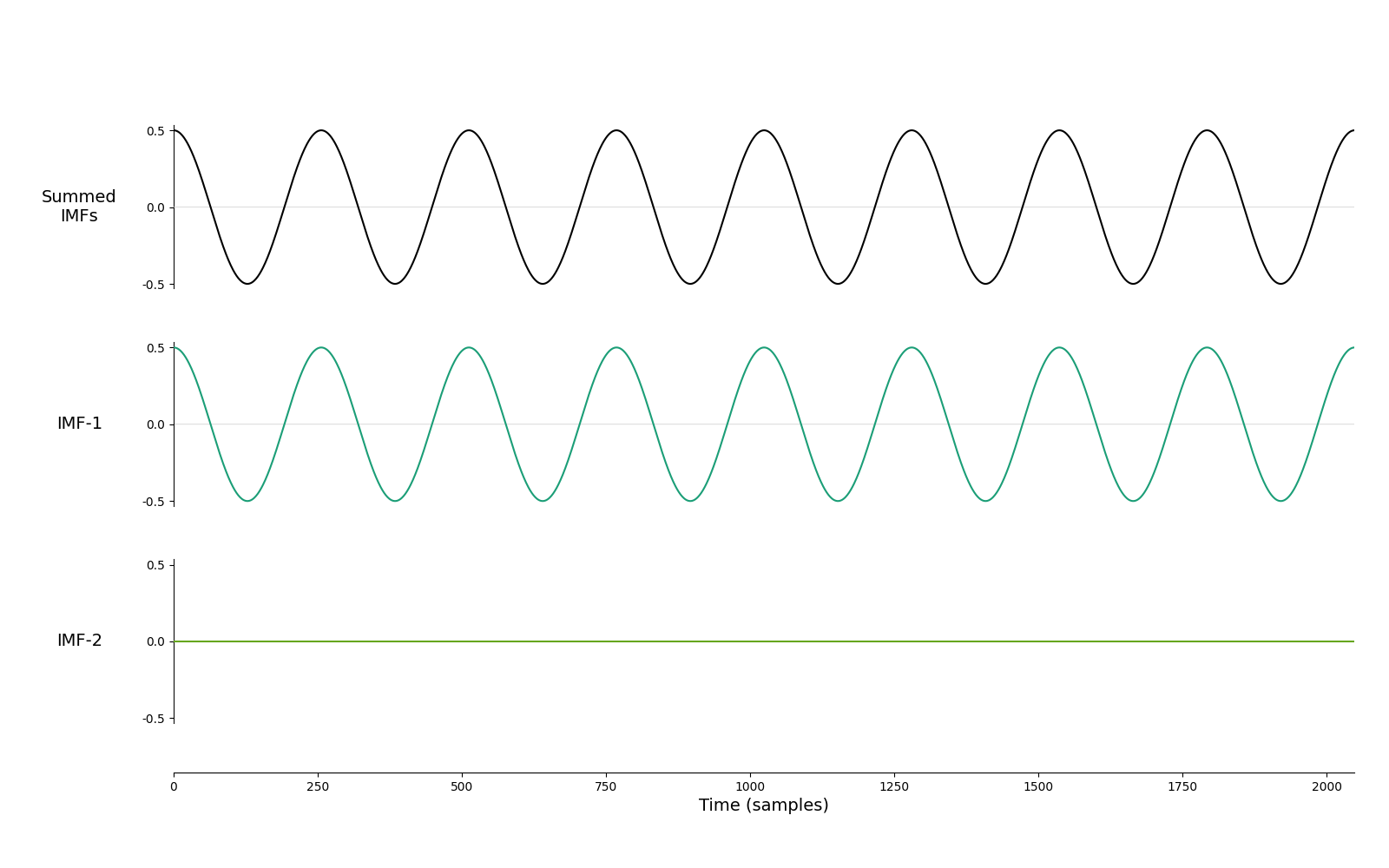<AxesSubplot:xlabel='Time (samples)'>


This is an easy decomposition as we haven’t added any noise to the signal. The oscillation is captured completed by the first component whilst the second component contains a very small residual.

Next we compute the EMD for the non-linear system

# Compute EMD
imf_nonlinear = emd.sift.sift(x_nonlinear)

# Visualise the IMFs
emd.plotting.plot_imfs(imf_nonlinear[:sample_rate*4, :])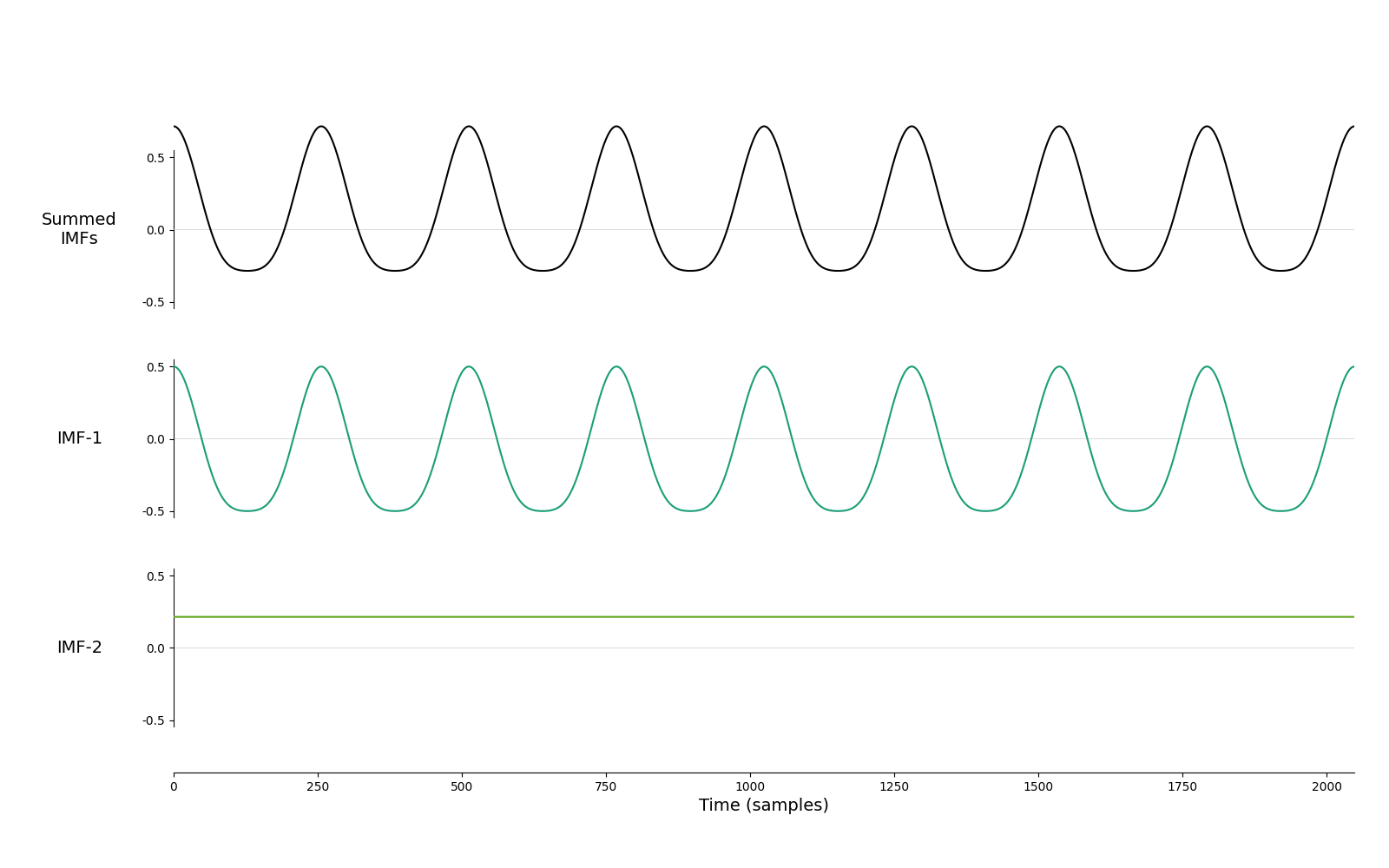<AxesSubplot:xlabel='Time (samples)'>


As with the linear system, this is an easy decomposition without any noise. The oscillatory signal is captured within the first component without further distorting the waveform shape. The residual contains a near-constant mean term. This is as the non-linear system makes the peaks larger and the troughs smaller which shifts the mean of the signal away from zero. This effect is often called rectification.

Next, we compute the instantanous frequency metrics from our linear and non-linear IMFs.

IP_linear, IF_linear, IA_linear = emd.spectra.frequency_transform(imf_linear, sample_rate, 'nht')
IP_nonlinear, IF_nonlinear, IA_nonlinear = emd.spectra.frequency_transform(imf_nonlinear, sample_rate, 'nht')


We can now start to look at how a non-sinusoidal waveform is represented in frequency. We will compare the EMD instantaneous frequency perspective with a standard frequency analysis based on the Fourier transform.

We compute the Hilbert-Huang transform from the IMF frequency metrics and Welch’s Periodogram from the raw data before creating a summary plot.

# Welch's Periodogram
fft_f, pxx_linear = signal.welch(x_linear, fs=sample_rate, nperseg=2048)
fft_f, pxx_nonlinear = signal.welch(x_nonlinear, fs=sample_rate, nperseg=2048)

# Hilbert-Huang transform
freq_range = (0, 20, 64)
hht_f, spec_linear = emd.spectra.hilberthuang(IF_linear, IA_linear, freq_range, scaling='density', sum_imfs=False)
hht_f, spec_nonlinear = emd.spectra.hilberthuang(IF_nonlinear, IA_nonlinear, freq_range, scaling='density', sum_imfs=False)

# Summary figure
plt.figure()
plt.subplot(121)
plt.plot(fft_f, pxx_linear)
plt.plot(fft_f, pxx_nonlinear)
plt.title("Welch's Periodogram")
plt.xlim(0, 20)
plt.xticks(np.arange(10)*2)
plt.grid(True)
plt.xlabel('Frequency (Hz)')

plt.subplot(122)
plt.plot(hht_f, spec_linear[:, 0])
plt.plot(hht_f, spec_nonlinear[:, 0])
plt.xticks(np.arange(10)*2)
plt.grid(True)
plt.title("Hilbert-Huang Transform")
plt.legend(['Linear System', 'Nonlinear System'])
plt.xlabel('Frequency (Hz)')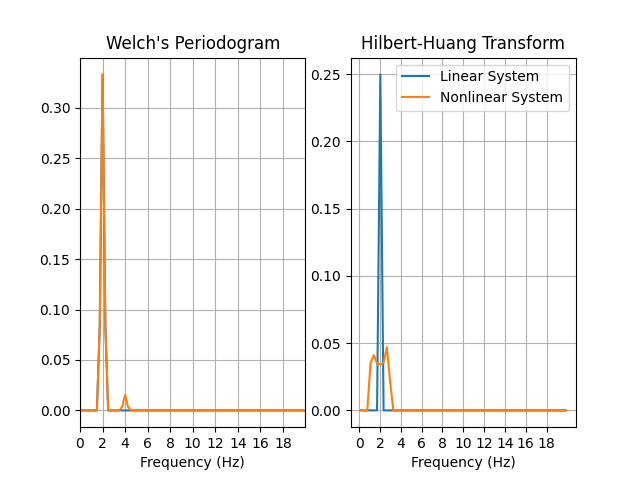Text(0.5, 23.52222222222222, 'Frequency (Hz)')


Both the Welch and Hilbert-Huang transform show a clear 2Hz peak for the linear system but differ in how the represent the non-linear system. Welch’s Periodogram introduces a harmonic component at 4Hz whereas the Hilbert-Huang transform simply widens the existing 2Hz peak.

Why would a non-sinsuoidal signal lead to a wider spectral peak in the Hilbert-Huang transform? To get some intuition about this, we will plot the Hilbert-Huang spectra alongside the instantaneous frequency traces for the linear and non-linear systems.

plt.figure(figsize=(10, 6))
plt.axes([.1, .1, .2, .8])
plt.plot(spec_linear, hht_f)
plt.plot(spec_nonlinear, hht_f)
plt.ylim(0, 10)
plt.ylabel('Frequency (Hz)')
plt.xlabel('Power')
plt.title('HHT')

plt.axes([.32, .1, .65, .8])
plt.plot(t, IF_linear[:, 0])
plt.plot(t, IF_nonlinear[:, 0])
plt.ylim(0, 10)
plt.xlim(0, 5)
plt.legend(['Linear system', 'Nonlinear-system'])
plt.title('Instantaneous Frequency')
plt.xlabel('Time (seconds)')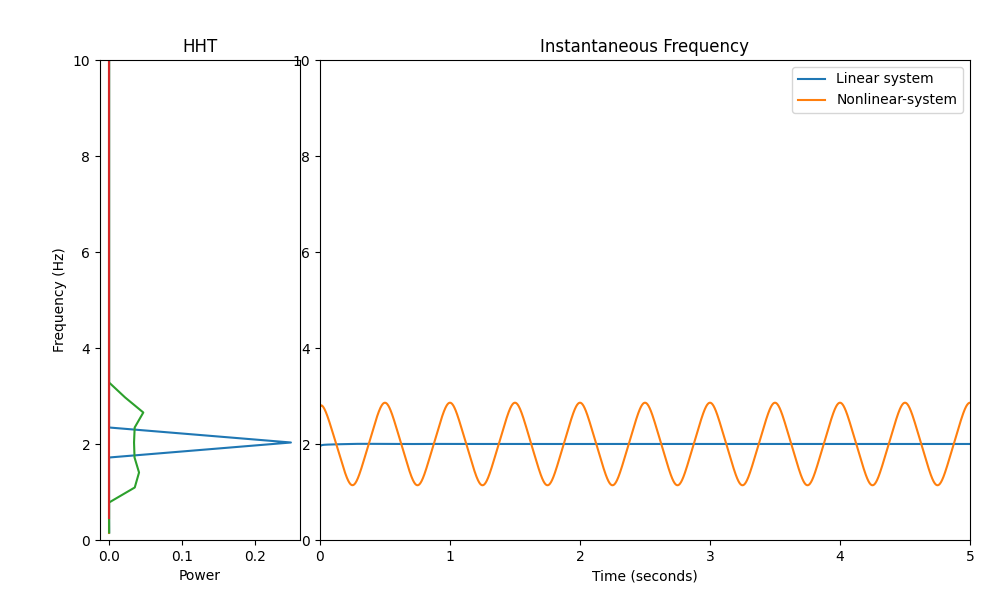Text(0.5, 30.72222222222222, 'Time (seconds)')


We see that the linear system has a constant instantaneous frequency which doesn’t vary over time. When this constant instantanous frequency is fed into the Hilbert-Huang transform it concentrates all the power into a sharp peak which looks similar to Welch’s periodogram.

In contrast, the instantanous frequency of the non-linear system does change over time. In fact, it seems to be oscillating between values aorund 2Hz (The IF variability is actually 2+/- the value for eta defined in the function above). When this variable instantaneous frequency is fed into the Hilbert-Huang transform, it spreads the power out within this same range.

If you re-run this analysis with a small value of eta in the nonlinear_system function you will see that the instantaneous frequency here varies within a smaller range and the peak in the Hilbert-Huang transform gets sharper again.

The variability in instantaneous frequency reflects the waveform shape distortions introduced by the non-linear system. We can see this by taking a look at the original waveform and the instantnaous freuqencies alongside each other.

plt.figure(figsize=(10, 8))
plt.subplot(211)
plt.plot(t, x_linear)
plt.plot(t, x_nonlinear)
plt.xlim(0, 3)
plt.ylabel('Signal\nAmplitude')
plt.subplot(212)
plt.plot(t, IF_linear[:, 0])
plt.plot(t, IF_nonlinear[:, 0])
plt.xlim(0, 3)
plt.ylim(0, 4)
plt.xlabel('Time (seconds)')
plt.ylabel('Instantaneous\nFrequency (Hz)')
plt.legend(['Linear system', 'Nonlinear-system'])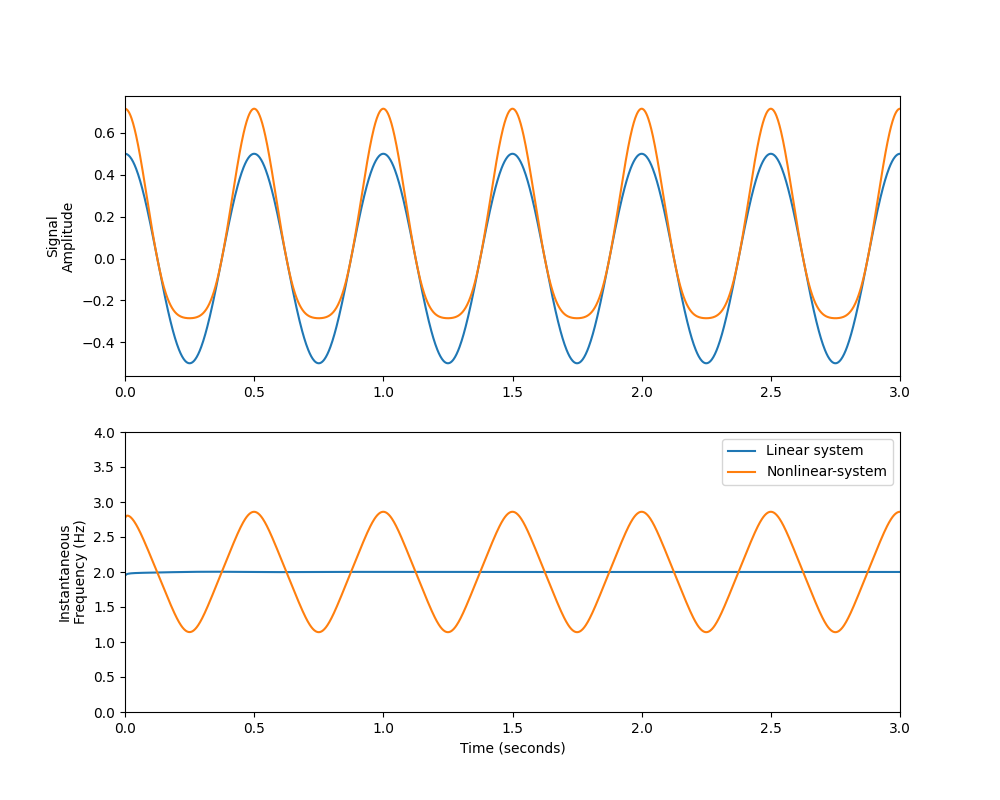<matplotlib.legend.Legend object at 0x7fbf44103e90>


The peaks in instantaneous frequency co-incide with the peaks of the raw signal, whilst the lowest instantaneous frequency values occur around the trough. This reflects how quickly the oscillation is progressing at each point in the cycle. The linear system progresses at a uniform rate throughout each cycle and therefore has a constant instantaneous frequency. In contrast, the sharp peaks and wide troughs of the non-linear signal can be interpreted as the cycle processing more quickly and slowly at the peak and trough respectively. The instantnaous frequency tracks this at the full sample rate of the data showing high frequnecies around the sharp peaks and low frequencies around the slow troughs.

## Single cycle Instantaneous Frequency#

We can extract the instantaneous frequency of single cycles of an oscillation to systematically compare differences in waveform shape between signals. Next we extract the indices of individual cycles in our systems and extract the IMF time-course waveform and instantaneous frequency of these cycles into separate matrices.

# Find cycle indices
cycles_linear = emd.cycles.get_cycle_vector(IP_linear, return_good=True)
cycles_nonlinear = emd.cycles.get_cycle_vector(IP_nonlinear, return_good=True)

# Pre-allocate an array for linear cycles
waveform_linear = np.zeros((300, cycles_linear.max()))*np.nan
instfreq_linear = np.zeros((300, cycles_linear.max()))*np.nan

# Extract waveform and IF info
for ii in range(1, cycles_linear.max()+1):
inds = cycles_linear[:, 0] == ii
waveform_linear[:np.sum(inds), ii-1] = imf_linear[inds, 0]
instfreq_linear[:np.sum(inds), ii-1] = IF_linear[inds, 0]

# Pre-allocate an array for non-linear cycles
waveform_nonlinear = np.zeros((300, cycles_nonlinear.max()))*np.nan
instfreq_nonlinear = np.zeros((300, cycles_nonlinear.max()))*np.nan

# Extract waveform and IF info
for ii in range(1, cycles_nonlinear.max()+1):
inds = cycles_nonlinear[:, 0] == ii
waveform_nonlinear[:np.sum(inds), ii-1] = imf_nonlinear[inds, 0]
instfreq_nonlinear[:np.sum(inds), ii-1] = IF_nonlinear[inds, 0]


We next directly compare waveform of examples cycles from the linear and non-linear systems. We plot the fifth cycle as an example. The first panel plots the waveform of the example cycles, there is a clear difference in waveform shape between the linear and non-linear systems. Panel 2 plots the instantaneous phase, the phase of the linear system progresses in a straight line whilst the nonlinear phase progresses at different rates throughout the cycle - it starts quickly but then slows. These dynamics are illustrated in panel 3 showing the instantaneous frequencies (computed from the derivative of the phase). The non-linear cycle has a relatively high frequency at the start of the cycle and a relatively low frequency at the end. In contrast, the linear cycle has a constant frequency.

cycle_ind_linear = cycles_linear[:, 0] == 5
cycle_ind_nonlinear = cycles_nonlinear[:, 0] == 5

plt.figure(figsize=(4, 8))
plt.subplot(311)
plt.plot(imf_linear[cycle_ind_linear, 0])
plt.plot(imf_nonlinear[cycle_ind_nonlinear, 0])
plt.xlabel('Samples')
plt.grid(True)

plt.subplot(312)
plt.plot(IP_linear[cycle_ind_linear, 0])
plt.plot(IP_nonlinear[cycle_ind_nonlinear, 0])
plt.ylabel('Instataneous\nPhase')
plt.xlabel('Samples')
plt.yticks(np.linspace(0, np.pi*2, 5), [r'$0$', r'$\pi/2$', r'$\pi$', r'$3\pi/2$', r'$2\pi$'])
plt.grid(True)

plt.subplot(313)
plt.plot(IF_linear[cycle_ind_linear, 0])
plt.plot(IF_nonlinear[cycle_ind_nonlinear, 0])
plt.ylabel('Instataneous\nFrequency')
plt.xlabel('Samples')
plt.grid(True)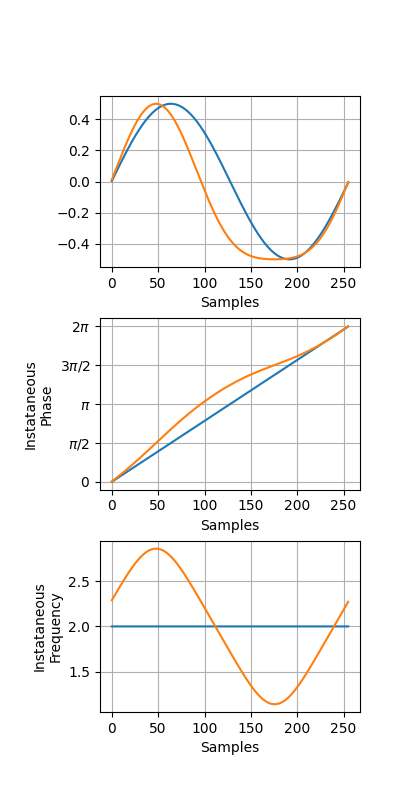Unfortuanetely, We cannot directly compare these two instantaneous frequency profiles as they are. Though both are the same length the change in waveform shape means that the x-axis is misaligned between them. Specifically, we can see that the nonlinear cycle has a descending zero-crossing around 100 samples into the cycle whilst the linear cycle crosses zero around 125 samples in. Similarly the peak and trough are a little shifted between the cycles.

As such, if we simply compute a comparison between these cycles, we cannot be sure we’re comparing featuers like for like. We may be contrasting the shoulder of one cycle with the zero-crossing of another.

One solution is called phase-alignment. We can plot the evolution of each cycle as over its phase rather than over time. We do this for the waveform and the instantaneous frequency next.

plt.figure(figsize=(10, 4))
plt.subplot(121)
plt.plot(IP_linear[cycle_ind_linear, 0], imf_linear[cycle_ind_linear, 0])
plt.plot(IP_nonlinear[cycle_ind_nonlinear, 0], imf_nonlinear[cycle_ind_nonlinear, 0])
plt.xlabel('Instataneous\nPhase')
plt.ylabel('Instataneous\nFrequency')
plt.xticks(np.linspace(0, np.pi*2, 5), [r'$0$', r'$\pi/2$', r'$\pi$', r'$3\pi/2$', r'$2\pi$'])
plt.grid(True)
plt.title('Phase-aligned\nWaveforms')

plt.subplot(122)
plt.plot(IP_linear[cycle_ind_linear, 0], IF_linear[cycle_ind_linear, 0])
plt.plot(IP_nonlinear[cycle_ind_nonlinear, 0], IF_nonlinear[cycle_ind_nonlinear, 0])
plt.xlabel('Instataneous\nPhase')
plt.ylabel('Instataneous\nFrequency')
plt.xticks(np.linspace(0, np.pi*2, 5), [r'$0$', r'$\pi/2$', r'$\pi$', r'$3\pi/2$', r'$2\pi$'])
plt.grid(True)
plt.title('Phase-aligned\nInst. Freq')Text(0.5, 1.0, 'Phase-aligned\nInst. Freq')


The phase-alignment makes the waveform of both cycles nearly exactly sinusoidal. Crucially information about the waveform shape of the cycles in the instantaneous frequency is preserved. We can see that at the cycle peak (where phase is pi/2) the nonlinear cycle has a much higher frequency than the linear cycle. The frequencies of the two cycles are nearly equal just after the ascending zerp-crossing (where phase is pi) and the nonlinear cycle has a much lower frequency around the trough.

This presentation of the data makes comparisons across cycles much simpler. We can now discuss differences between the shape of specific parts of a cycle - such as the peak or trough.

## A dynamic oscillation with noise#

Unfortunately most signals are more complex than our sine-wave above! Here we apply the same analysis as above to a dynamic & noisy signal. The signal dynamics make the signal more interesting but also introduce some challenges for waveform shape analyses, we will explore what these are and how phase-alignment can be useful to overcome them.

First we generate a dynamic oscillation using direct pole-placement to create an autoregressive model with a peak around 15Hz. We then pass the dynamic oscillation through our linear and non-linear systems as above. Finally we add some white noise.

x = x * 1e-5
peak_freq = 15
sample_rate = 256
seconds = 10
noise_std = None
x = emd.simulate.ar_oscillator(peak_freq, sample_rate, seconds,
noise_std=noise_std, random_seed=42, r=.96) / 150
t = np.linspace(0, seconds, seconds*sample_rate)

x_linear = linear_system(x, K=1) + np.random.randn(len(t), 1)*0.5
x_nonlinear = nonlinear_system(x, K=1, eta=0.25) + np.random.randn(len(t), 1)*0.5


We compute our IMFs using the iterated_mask_sift with default parameters. First on the linear system.

# Compute IMFs

# Visualise IMFs
emd.plotting.plot_imfs(imf_linear[:sample_rate*4, :])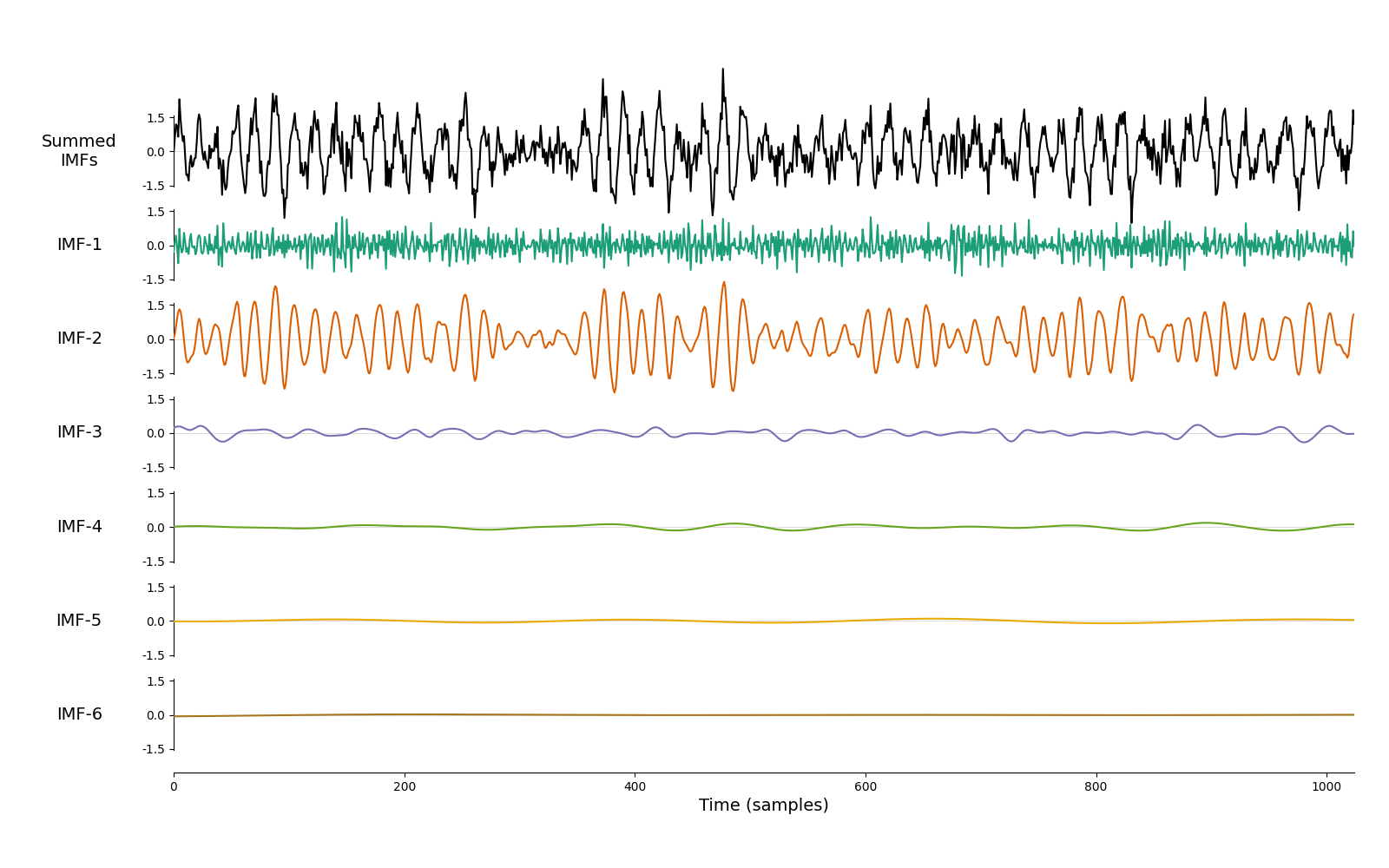<AxesSubplot:xlabel='Time (samples)'>


The oscillation is isolated into IMF-2. The remaining IMFs comtain low magnitude noise. Next we run the same on the non-linear system.

# Compute IMFs

# Visualise IMFs
emd.plotting.plot_imfs(imf_nonlinear[:sample_rate*4, :])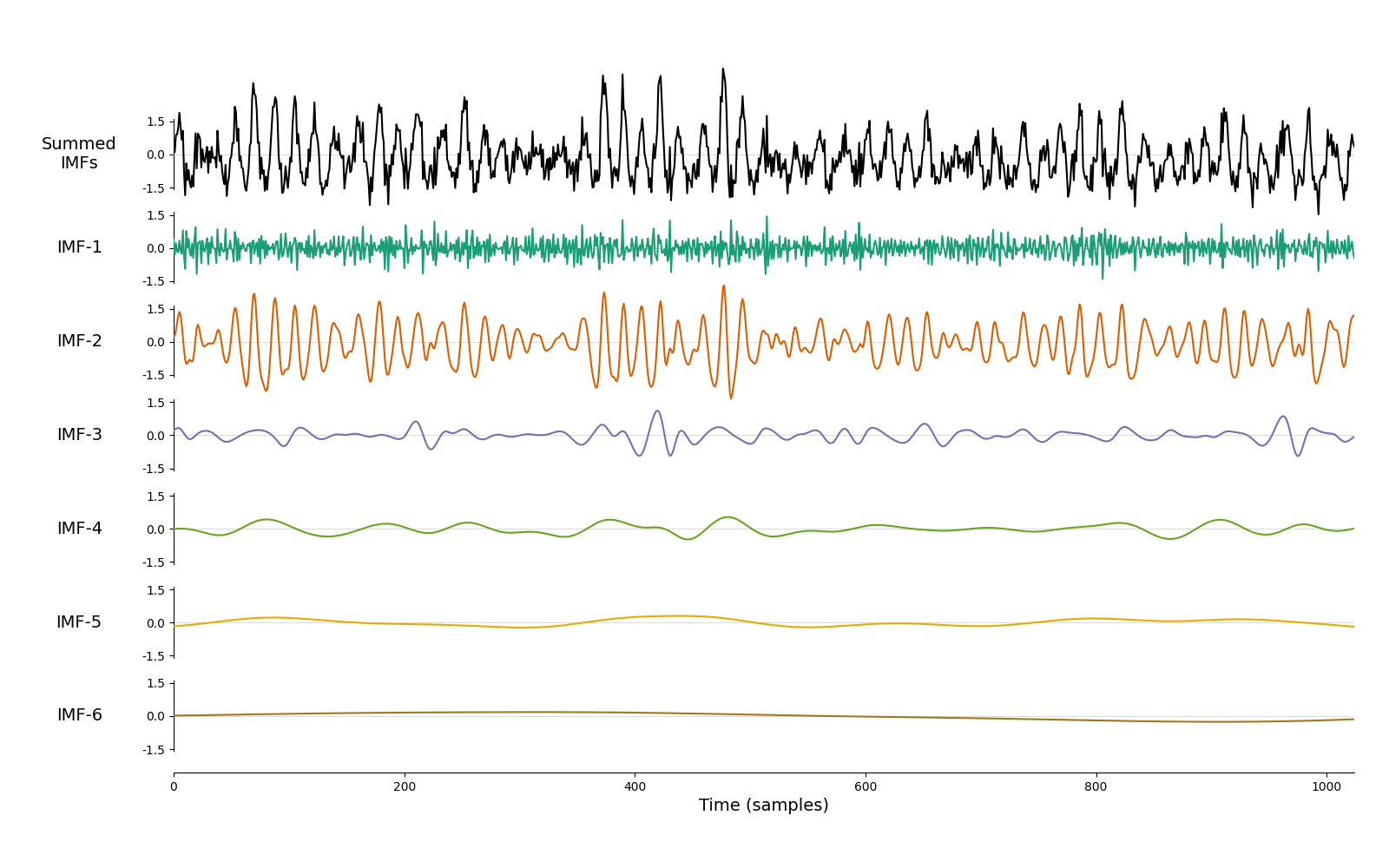<AxesSubplot:xlabel='Time (samples)'>


Again the oscillatory component is isolated into IMF-2. Next we compute the instantanous frequency metrics for the linear and nonlinear IMFs using the Normalise Hilbert Transform.

IP_linear, IF_linear, IA_linear = emd.spectra.frequency_transform(imf_linear, sample_rate, 'nht')
IP_nonlinear, IF_nonlinear, IA_nonlinear = emd.spectra.frequency_transform(imf_nonlinear, sample_rate, 'nht')


We next compare the spectral content of the signal using the EMD based Hilbert-Huang transform and the Fourier based Welch’s Periodogram.

# Welch's Periodogram
fft_f, pxx_linear = signal.welch(x_linear[:, 0], fs=sample_rate, nperseg=2048)
fft_f, pxx_nonlinear = signal.welch(x_nonlinear[:, 0], fs=sample_rate, nperseg=2048)

# Hilbert-Huang Transform
freq_range = (0, 40, 64)
hht_f, spec_linear = emd.spectra.hilberthuang(IF_linear, IA_linear, freq_range, mode='amplitude', sum_imfs=False)
hht_f, spec_nonlinear = emd.spectra.hilberthuang(IF_nonlinear, IA_nonlinear, freq_range, mode='amplitude', sum_imfs=False)

# Summary figure
plt.figure()
plt.subplot(121)
plt.plot(fft_f, pxx_linear)
plt.plot(fft_f, pxx_nonlinear)
plt.title("Welch's Periodogram")
plt.xlabel('Frequency (Hz)')
plt.ylabel('Power')
plt.xlim(0, 40)
plt.subplot(122)
plt.plot(hht_f, spec_linear[:, 1])
plt.plot(hht_f, spec_nonlinear[:, 1])
plt.xlabel('Frequency (Hz)')
plt.title("Hilbert-Huang Transform")
plt.legend(['Linear System', 'Nonlinear System'])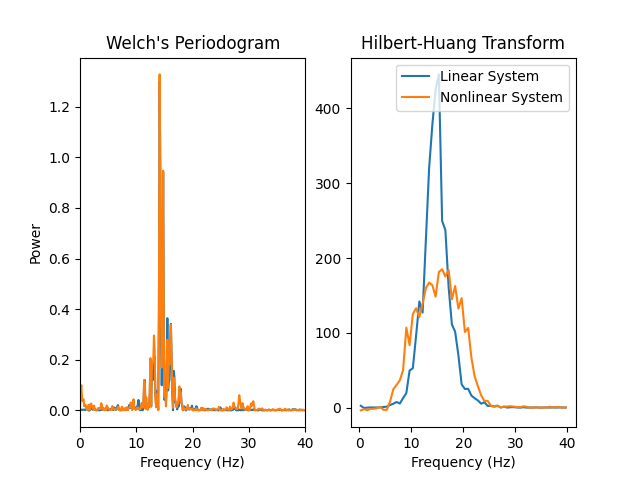<matplotlib.legend.Legend object at 0x7fbf4109b810>


As with the simple sinusoidal signal in the first section. We see that the non-sinsusoidal waveform introduced by the nonlinear system introduces a harmonic into Welch’s Periodogram and widens the 15Hz peak of the Hilbert-Huang transform.

We can plot the waveform and instantanous frequency alongside each other to try and see how the shape might be affecting instantanous frequency.

plt.figure(figsize=(8, 6))
plt.subplot(211)
plt.plot(t, imf_linear[:, 1])
plt.plot(t, imf_nonlinear[:, 1])
plt.xlim(0, 3)
plt.subplot(212)
plt.plot(t, IF_linear[:, 1])
plt.plot(t, IF_nonlinear[:, 1])
plt.xlim(0, 3)
plt.ylim(0, 25)
plt.ylabel('Instantaneous\nFrequency (Hz)')
plt.xlabel('Time (seconds)')
plt.legend(['Linear System', 'Nonlinear System'])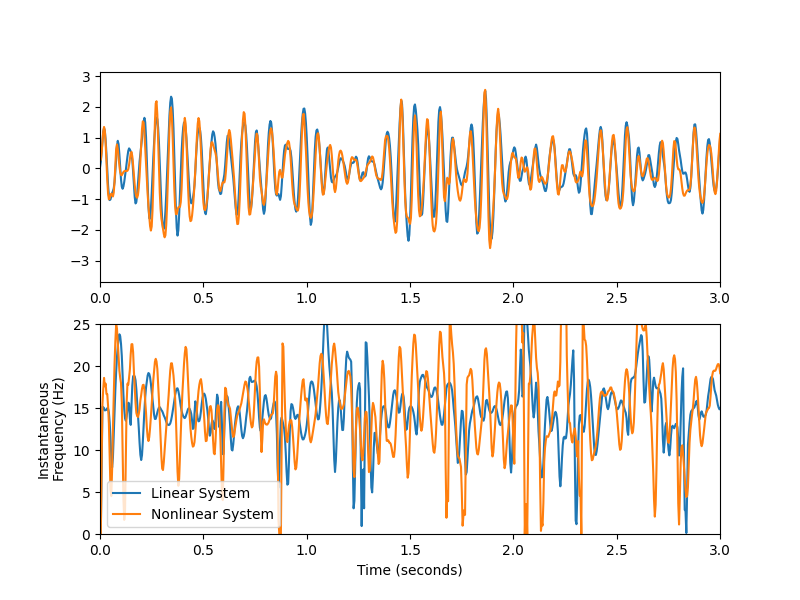<matplotlib.legend.Legend object at 0x7fbf40f9d490>


In contrast to the simple sinusoidal case, this plot looks very noisy. The instantaneous frequency estimates are very volitile in parts of the signal with low amplitude (such as 1-1.75 seconds). If we concentrate on clean parts of the signal (say 0-0.5 seconds) we can perhaps see a suggestion that the non-linear instantnaous frequency is changing more than the linear one but it is perhaps hard to tell from this alone.

We can try to clean up the analysis by contentrating on oscillatory cycles which have a well formed phase and an amplitude above a specified threshold. We extract these cycles using the emd.cycles.get_cycle_vector function with a defined mask based on instantanous amplitude. We apply a mask here as phase estimates, and therefore instantaneous frequency estimates, can be very noisy in very low power signals - including the very low amplitude cycles we could get noisy frequency jumps which will distort our average. This mask removes around 20% of the cycles with the vary lowest amplitudes to avoid this noise.

mask = IA_linear[:, 1] > .05

mask = IA_nonlinear[:, 1] > .05


Next we extract the per-cycle IMF and instantaneous frequencies for both systems. We will also compute the ‘control points’ for each cycle, these are the indices of the peak, zero-crossings and trough of each cycle.

waveform_linear = np.zeros((30, cycles_linear.max()))*np.nan
instfreq_linear = np.zeros((30, cycles_linear.max()))*np.nan

for ii in range(1, cycles_linear.max()+1):
inds = cycles_linear[:, 1] == ii
waveform_linear[:np.sum(inds), ii-1] = imf_linear[inds, 1]
instfreq_linear[:np.sum(inds), ii-1] = IF_linear[inds, 1]

ctrl_linear = emd.cycles.get_control_points(imf_linear[:, 1], cycles_linear[:, 1])

waveform_nonlinear = np.zeros((30, cycles_nonlinear.max()))*np.nan
instfreq_nonlinear = np.zeros((30, cycles_nonlinear.max()))*np.nan

for ii in range(1, cycles_nonlinear.max()+1):
inds = cycles_nonlinear[:, 1] == ii
waveform_nonlinear[:np.sum(inds), ii-1] = imf_nonlinear[inds, 1]
instfreq_nonlinear[:np.sum(inds), ii-1] = IF_nonlinear[inds, 1]

ctrl_nonlinear = emd.cycles.get_control_points(imf_nonlinear[:, 1], cycles_nonlinear[:, 1])


Next we plot a big summary of the single cycle IMFs. The top row contains each single cycle and the second row contains the average across cycles. As with the simple oscillation in the first example, we can see by eye that the variability in the signal is shifting the relative timing of the peaks and troughs within each cycle.

Unfortunately there is a second problem caused by the dynamics in this signal. As well as shape induced variability in the timinig of features within cycles, there is also variability in the overall durations of each cycle. These both add up to create considerable noise in cross cycle comparisons.

The third row contains histograms of the timings of the control points within each cycle. The further we get from the ‘locking-point’ at the start of the cycle, the wider these distributions become. The troughs can occur anywhere between 25 and 40 samples into a cycle.

Finally the fourth row show the number of cycles contributing to the average at each point in time. After 40 samples there are relatively few cycles long enough to contribute to an average at this point. Around 50-60 cycles we have almost none.

plt.figure(figsize=(6, 9))
plt.subplot(421)
plt.title('Linear oscillations')
plt.plot(waveform_linear)
plt.xticks(np.arange(5)*10, [])
plt.grid(True)

plt.subplot(422)
plt.title('Nonlinear oscillations')
plt.plot(waveform_nonlinear)
plt.xticks(np.arange(5)*10, [])
plt.grid(True)

plt.subplot(423)
plt.title('Linear avg. waveform')
plt.plot(np.nanmean(waveform_linear, axis=1))
plt.xticks(np.arange(5)*10, [])
plt.grid(True)

plt.subplot(424)
plt.title('Nonlinear avg. waveform')
plt.plot(np.nanmean(waveform_nonlinear, axis=1))
plt.xticks(np.arange(5)*10, [])
plt.grid(True)

plt.subplot(425)
plt.title('Linear ctrl points')
plt.hist(ctrl_linear[:, 1], np.linspace(0, 40))
plt.hist(ctrl_linear[:, 2], np.linspace(0, 40))
plt.hist(ctrl_linear[:, 3], np.linspace(0, 40))
plt.xticks(np.arange(5)*10, [])
plt.grid(True)

plt.subplot(426)
plt.title('Nonlinear ctrl points')
plt.hist(ctrl_nonlinear[:, 1], np.linspace(0, 40))
plt.hist(ctrl_nonlinear[:, 2], np.linspace(0, 40))
plt.hist(ctrl_nonlinear[:, 3], np.linspace(0, 40))
plt.xticks(np.arange(5)*10, [])
plt.grid(True)
plt.legend(['Peak', 'Desc ZC', 'Trough'])

plt.subplot(427)
plt.title('Linear Num Cycles')
plt.plot(np.sum(~np.isnan(waveform_linear), axis=1))
plt.xticks(np.arange(5)*10)
plt.grid(True)

plt.subplot(428)
plt.xlabel('Samples')
plt.title('Nonlinear Num Cycles')
plt.plot(np.sum(~np.isnan(waveform_nonlinear), axis=1))
plt.xticks(np.arange(5)*10)
plt.grid(True)/home/docs/checkouts/readthedocs.org/user_builds/emd/checkouts/stable/doc/source/tutorials/03_cycle_ananlysis/emd_tutorial_03_cycle_04_waveform.py:558: RuntimeWarning: Mean of empty slice
plt.plot(np.nanmean(waveform_linear, axis=1))
/home/docs/checkouts/readthedocs.org/user_builds/emd/checkouts/stable/doc/source/tutorials/03_cycle_ananlysis/emd_tutorial_03_cycle_04_waveform.py:564: RuntimeWarning: Mean of empty slice
plt.plot(np.nanmean(waveform_nonlinear, axis=1))


We can make the equivalent plot for the instantaneous frequency - again the two sources in variability are visible.

plt.figure(figsize=(6, 9))
plt.subplot(421)
plt.title('Linear oscillations')
plt.plot(instfreq_linear)
plt.xticks(np.arange(5)*10, [])
plt.grid(True)

plt.subplot(422)
plt.title('Nonlinear oscillations')
plt.plot(instfreq_nonlinear)
plt.xticks(np.arange(5)*10, [])
plt.grid(True)

plt.subplot(423)
plt.title('Linear avg. instfreq')
plt.plot(np.nanmean(instfreq_linear, axis=1))
plt.xticks(np.arange(5)*10, [])
plt.grid(True)

plt.subplot(424)
plt.title('Nonlinear avg. instfreq')
plt.plot(np.nanmean(instfreq_nonlinear, axis=1))
plt.xticks(np.arange(5)*10, [])
plt.grid(True)

plt.subplot(425)
plt.title('Linear ctrl points')
plt.hist(ctrl_linear[:, 1], np.linspace(0, 40))
plt.hist(ctrl_linear[:, 2], np.linspace(0, 40))
plt.hist(ctrl_linear[:, 3], np.linspace(0, 40))
plt.xticks(np.arange(5)*10, [])
plt.grid(True)

plt.subplot(426)
plt.title('Nonlinear ctrl points')
plt.hist(ctrl_nonlinear[:, 1], np.linspace(0, 40))
plt.hist(ctrl_nonlinear[:, 2], np.linspace(0, 40))
plt.hist(ctrl_nonlinear[:, 3], np.linspace(0, 40))
plt.xticks(np.arange(5)*10, [])
plt.grid(True)
plt.legend(['Peak', 'Desc ZC', 'Trough'])

plt.subplot(427)
plt.title('Linear Num Cycles')
plt.plot(np.sum(~np.isnan(instfreq_linear), axis=1))
plt.xticks(np.arange(5)*10)
plt.grid(True)

plt.subplot(428)
plt.xlabel('Samples')
plt.title('Nonlinear Num Cycles')
plt.plot(np.sum(~np.isnan(instfreq_nonlinear), axis=1))
plt.xticks(np.arange(5)*10)
plt.grid(True)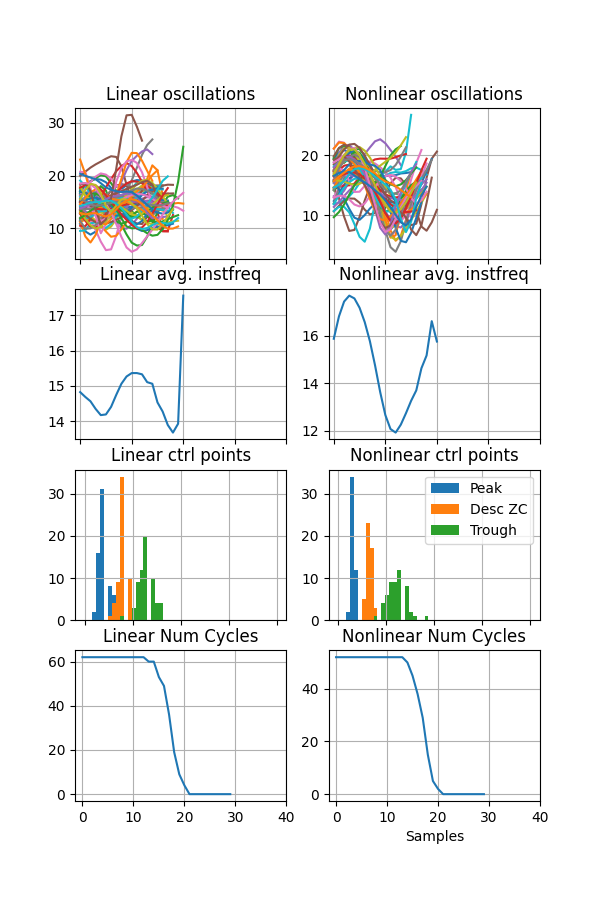/home/docs/checkouts/readthedocs.org/user_builds/emd/checkouts/stable/doc/source/tutorials/03_cycle_ananlysis/emd_tutorial_03_cycle_04_waveform.py:618: RuntimeWarning: Mean of empty slice
plt.plot(np.nanmean(instfreq_linear, axis=1))
/home/docs/checkouts/readthedocs.org/user_builds/emd/checkouts/stable/doc/source/tutorials/03_cycle_ananlysis/emd_tutorial_03_cycle_04_waveform.py:624: RuntimeWarning: Mean of empty slice
plt.plot(np.nanmean(instfreq_nonlinear, axis=1))


We can see an indication that there may be a difference in shape between the linear and nonlinear systems but it is hard to interpret when looking at the waveform or instantaneous frequency as a function of time.

As in the first section, we can make a cleaner comparison considering the evolution of a cycle as a function of its phase rather than across time. Next we compute this phase alignment using emd.cycles.phase_align. This interpolates the instantaneous phase of a signal onto a regularly sampled grid before applying that interpolation onto a metric of our choice. Here we will phase align the IMF and the instantaneous frequency values for the linear and non linear systems.

pa_waveform_linear, phase_template = emd.cycles.phase_align(IP_linear[:, 1],
imf_linear[:, 1],
cycles=cycles_linear[:, 1])
pa_waveform_nonlinear, _ = emd.cycles.phase_align(IP_nonlinear[:, 1],
imf_nonlinear[:, 1],
cycles=cycles_nonlinear[:, 1])

pa_if_linear, _ = emd.cycles.phase_align(IP_linear[:, 1],
IF_linear[:, 1],
cycles=cycles_linear[:, 1])
pa_if_nonlinear, _ = emd.cycles.phase_align(IP_nonlinear[:, 1],
IF_nonlinear[:, 1],
cycles=cycles_nonlinear[:, 1])


Plotting the phase aligned results, we see that the IMF waveforms are transformed into near perfect sinusoids confirming that the phase alignment has done a good job at projecting the phase onto a regular grid.

When applying this projection onto the instantaneous frequencies we can see that the nonlinear cycle has a higher frequency peak and lower frequency trough than the linear cycles. Thanks to the phase alignment we can be confident that we are really comparing features like-for-like between the two system despite variability in timing of features within cycles and variability in the absolution duration of cycles.

plt.figure(figsize=(10, 4))
plt.subplot(121)
plt.plot(phase_template, pa_waveform_linear.mean(axis=1))
plt.plot(phase_template, pa_waveform_nonlinear.mean(axis=1))
plt.xlabel('Instataneous\nPhase')
plt.xticks(np.linspace(0, np.pi*2, 5), [r'$0$', r'$\pi/2$', r'$\pi$', r'$3\pi/2$', r'$2\pi$'])
plt.grid(True)
plt.title('Phase-aligned\nIMF')

plt.subplot(122)
plt.plot(phase_template, pa_if_linear.mean(axis=1))
plt.plot(phase_template, pa_if_nonlinear.mean(axis=1))
plt.ylim(10, 20)
plt.legend(['Linear System', 'Nonlinear system'])
plt.xlabel('Instataneous\nPhase')
plt.ylabel('Instataneous\nFrequency')
plt.xticks(np.linspace(0, np.pi*2, 5), [r'$0$', r'$\pi/2$', r'$\pi$', r'$3\pi/2$', r'$2\pi$'])
plt.grid(True)
plt.title('Phase-aligned\nInst. Freq')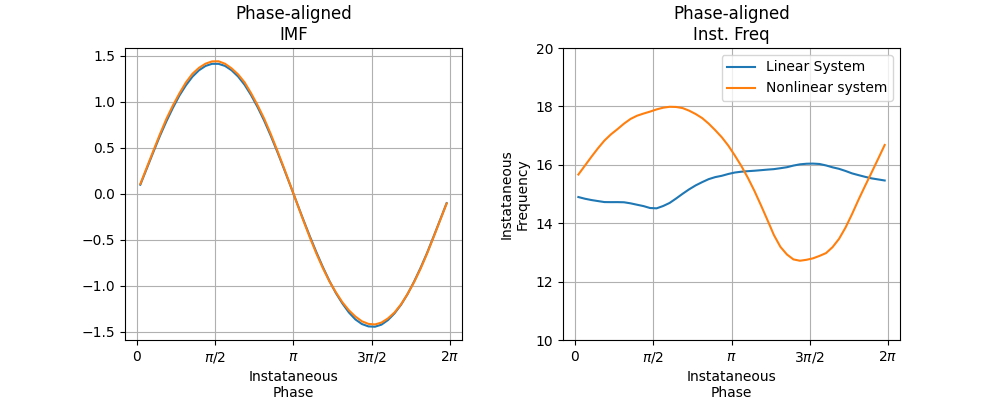Text(0.5, 1.0, 'Phase-aligned\nInst. Freq')


We can now use conventional statistics to compare waveforms between groups. Here we do an independent samples t-test to describe differences in phase aligned instantaneous frequency between the linear and nonlinear systems.

tvals, pvals = stats.ttest_ind(pa_if_nonlinear, pa_if_linear, axis=1)

plt.figure(figsize=(10, 4))
plt.subplot(121)
plt.plot(phase_template, pa_if_linear.mean(axis=1))
plt.plot(phase_template, pa_if_nonlinear.mean(axis=1))
plt.ylim(10, 20)
plt.legend(['Linear System', 'Nonlinear system'])
plt.xlabel('Instataneous\nPhase')
plt.ylabel('Instataneous\nFrequency')
plt.xticks(np.linspace(0, np.pi*2, 5), [r'$0$', r'$\pi/2$', r'$\pi$', r'$3\pi/2$', r'$2\pi$'])
plt.grid(True)
plt.title('Phase-aligned\nInst. Freq')

plt.subplot(122)
plt.plot(phase_template, tvals)
plt.xlabel('Instataneous\nPhase')
plt.ylabel('t-statistic')
plt.xticks(np.linspace(0, np.pi*2, 5), [r'$0$', r'$\pi/2$', r'$\pi$', r'$3\pi/2$', r'$2\pi$'])
plt.grid(True)
plt.title('Nonlinear > Linear IF')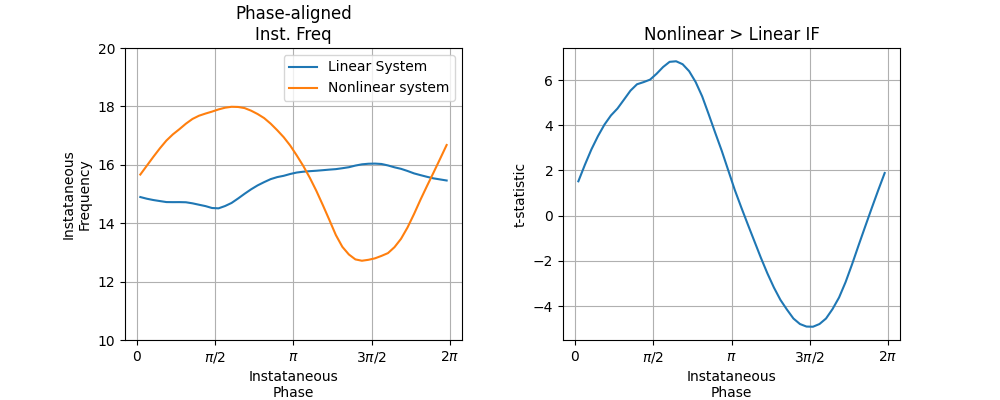Text(0.5, 1.0, 'Nonlinear > Linear IF')